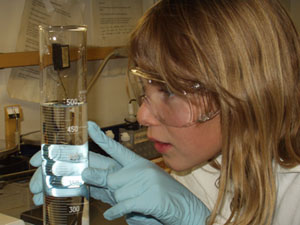# How to Integrate Measurement and Uncertainty

There are three distinct steps to integrating effective measurement technique into science instruction, and this module contains material and examples for each step.

## Step1: Teach the basic elements of effective measurement

This module contains student handout packets that can be used as instructional material to teach these concepts. These documents can be used to teach students the concepts of measurement uncertainty, how to determine the uncertainty of any measurement they make, and the uncertainty of any value they calculate using measured values. Packets include text, images, and examples, as well as conceptual and quantitative questions to build understanding of the material. There are two packets, each aimed at students at different academic levels:

##Step 2: Do activities to teach effective measurement

Hands-on lab activities help students try the methods in the packets using measurements they make, and solidify student understanding of effective measurement technique. These activities are specifically intended to teach effective measurement consistent with the approach outlined above:

## Step 3: Integrate effective measurement into existing lab activities

We provide some examples of how to convert labs so they are consistent with the methods described here. Science labs often have students compare their answers to "the actual value." This gives a false impression. First, it inevitably shows students that their answer is wrong. Second, it implies that science has correct answers, rather than measurements that are subject to some level of uncertainty. A method more consistent with the approach to measurement describe here is:
a. Students determine the uncertainty of each measurement they make in the lab
b. Students determine the uncertainty of numbers they calculate using their measurements
c. Students present their results as a range of possible values and compare this range with accepted values including their ranges of uncertainty OR
d. Students present their results in the form of a prediction – again as a range of possible values. They test their prediction to see whether they are correct within their stated range of certainty

Examples of this type of lab include: